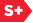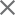### Proceedings Paper

Local inversion of the radon transform in the plane using wavelets
Author(s): David Walnut
Format Member Price Non-Member Price
PDF \$17.00 \$21.00

Paper Abstract

We use the theory of the continuous wavelet transform to derive inversion formulas for the Radon transform. These inversion formulas are local in even dimensions in the following sense. In order to recover a function f from its Radon transform in a ball of radius R > 0 about a point x to within error (epsilon) > 0, we can find (alpha) ((epsilon) ) > 0 such that this can be accomplished by knowing the projections of f only on lines passing through a ball of radius R + (alpha) ((epsilon) ) about x. We give explicit a priori estimates on the error in the L2 and L(infinity ) norms.

Paper Details

Date Published: 1 November 1993
PDF: 7 pages
Proc. SPIE 2034, Mathematical Imaging: Wavelet Applications in Signal and Image Processing, (1 November 1993); doi: 10.1117/12.162090
Show Author Affiliations
David Walnut, George Mason Univ. (United States)

Published in SPIE Proceedings Vol. 2034:
Mathematical Imaging: Wavelet Applications in Signal and Image Processing
Andrew F. Laine, Editor(s)